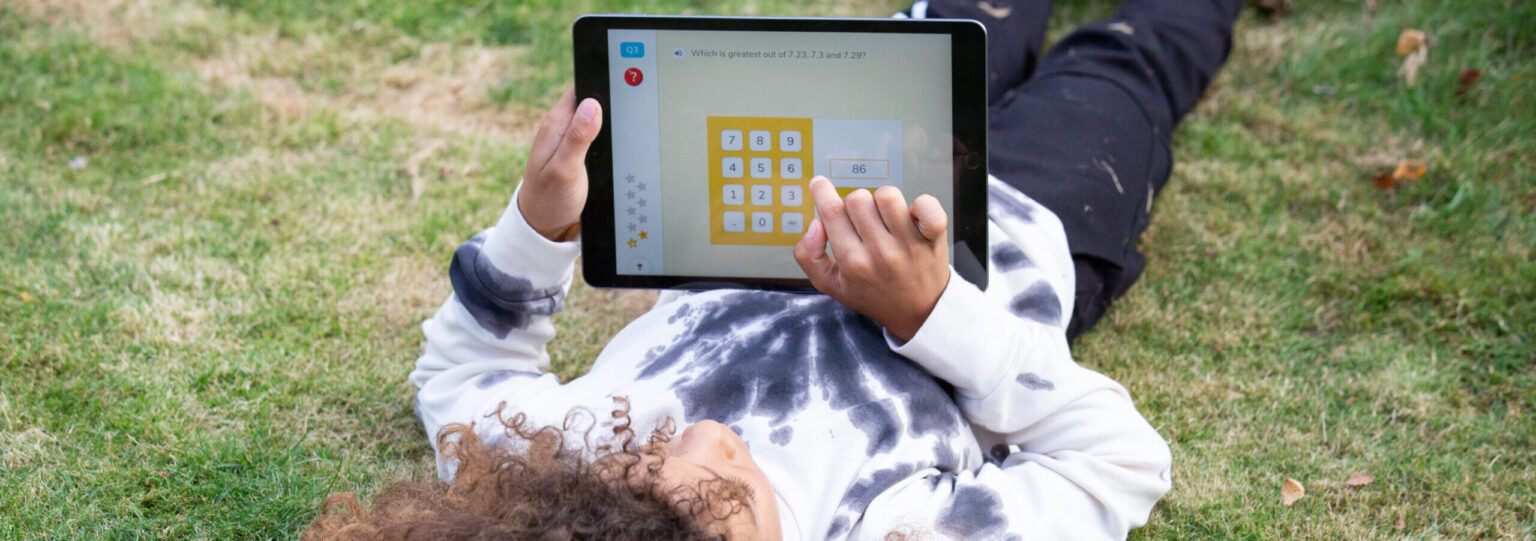# Prime numbers### Do you know these prime numbers facts?

1) What is the only even prime number? 2) What is the first prime number with consecutive digits? 3) Apart from 2, prime numbers always end in the same four digits – what are they? 4) A prime quadruplet is four prime numbers as close together as they can be within a decade. The first such example is 11, 13, 17, 19. What is the next example? 5) A palindromic prime reads the same forwards as backwards. The first three digit palindromic prime is 101. What is the next?

1) The only even prime number is 2. As you may well have worked out, all other even numbers have 2 as a factor.

2) The first prime number with consecutive digits is 23. This is one reason amongst many that some people believe that 23 is special (in fact, there’s even a group called the 23rdians who believe it has mystical powers). In maths, and particularly prime numbers, it has some unusual properties, for example, 11,111,111,111,111,111,111,111 (there are 23 number 1’s here) is a prime number 10^23 – 23 = 99,999,999,999,999,999,999,977 which is the largest 23 digit prime And in science, to create human life, each parent has to contribute 23 chromosomes, and the Earth is currently tilted at 23 degrees…

3) Since primes have to be odd (apart from 2) and cannot be a multiple of 5, they must always end in 1, 3, 7 or 9.

4) 101, 103, 107, 109 is the next one. Incidentally, if you cross decades, prime quintuplets and sextuplets also exist. Incredibly, 43777, 43781, 43783, 43787, 43789, 43793 are all prime.

5) The next is 131. Their search can rapidly be narrowed by a process of elimination. For example, we can immediately discount palindromic numbers in the 200’s since they will also end in 2 and thus be even. And we can discount 4-digit, 6-digit, 8-digit palindromes (etc.) because these can all be shown to be multiples of 11. So the next few are: 151, 181, 191, 313, 353, 373, 383, 727, 757, 787, 797, 919, 929, 10301, 10501, 10601, 11311, for example.

,

Related posts

# Are you a parent, teacher or student?

Are you a parent or teacher?

## Hi there!

Book a chat with our team